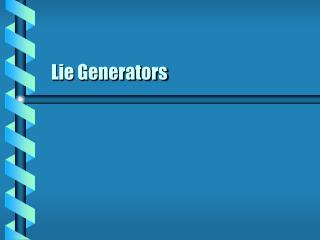DownloadDownload PresentationLie Generators

# Lie Generators

Télécharger la présentation## Lie Generators

- - - - - - - - - - - - - - - - - - - - - - - - - - - E N D - - - - - - - - - - - - - - - - - - - - - - - - - - -
##### Presentation Transcript

1. Lie Generators

2. Lie groups are continuous. Continuous coordinate system Finite dimension Origin is identity The multiplication law is by analytic functions. Two elements x, y Consider z = xy There are N analytic functions that define the coordinates. Based on 2N coordinates Lie Group Operation

3. The general linear groups GL(n, R) are Lie groups. Represent transformations Dimension is n2 All Lie groups are isomorphic to subgroups of GL(n, R). Example Let x, y  GL(n, R). Coordinates are matrix elements minus dab Find the coordinates of z=xy. Analytic in coordinates GL as Lie Group

4. Transformed Curves • All Lie groups have coordinate systems. • May define differentiable curves • The set x(e) may also form a group. • Subgroup g(e)

5. Parameterizations of subgroups may take different forms. Example Consider rotations about the Euclidean x-axis. May use either angle or sine The choice gives different rules for multiplication. Single-axis Rotation

6. One Parameter • A one-parameter subgroup can always be written in a standard form. • Start with arbitrary represenatation • Differentiable function m • Assume that there is a parameter • The differential equation will have a solution. • Invert to get parameter S1

7. The standard form can be used to find a parameter a independent of e. Solve the differential equation. The matrix a is an infinitessimal generator of g(e) Transformation Generator Using standard form next